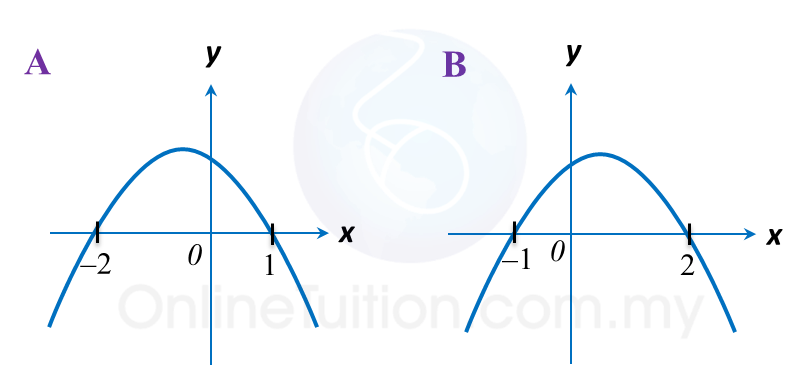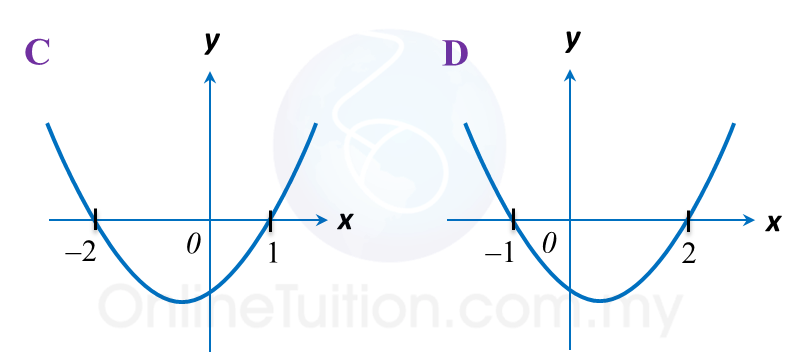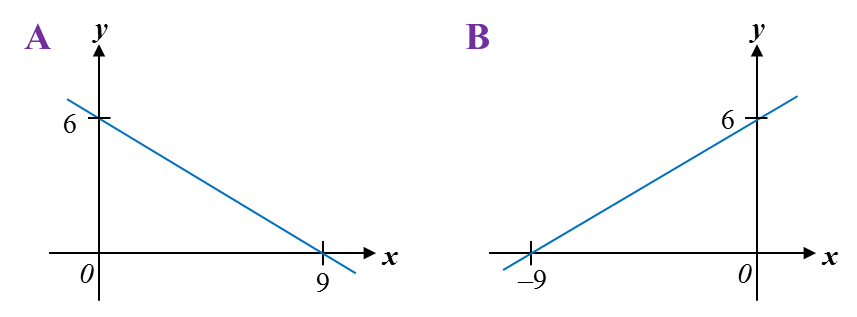# 2.4.1 Graph of Functions (II), SPM Paper 1 (Short Questions)

2.4 SPM Practice (Short Questions)

Question 1:
Which of the following graphs represent y = –x– x + 2?Solution:
1.   The coefficient of x2 < 0, its shape is ∩.
2.   Find the x-intercepts by substituting y = 0 into the function.
y = –x2x + 2
when y = 0
0 = (–x + 1) (x + 2)
x = 1, –2

Question 2:
Which of the following graphs represent 3y = 18 + 2x?Solution:
1.   The highest power of x = 1, it is a linear graph, i.e. a straight line.
2.   The coefficient of x > 0, the straight line is /.

3y = 18 + 2x
On the y-axis, x = 0.
3y = 18
y = 6

On the x-axis, y = 0.
0 = 18 + 2x
2x = –18
x = –9